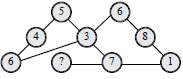# User ForumSubject :IMO    Class : Class 5

Which number will replace the question mark in the given number bond?A7
B8
C6
D5

How do u do this

## Ans 1:

Class : Class 8
Sum of number in each chain is 15.

## Ans 2:

Class : Class 5
SUM OF ALL CHAIN IS 15 @ ANSWER A YAY MINE IS RIGHT.

Class : Class 5

Class : Class 5

## Ans 5:

Class : Class 8
sum of all numbers in a line is 15 6 4 5=15, 6 3 6=15, 6 8 1=15,7 5 3=15 so Answer is A